Select Page

# Three Dimensional Geometry MCQ CBSE Maths 12 Science Answers in English

Three Dimensional Geometry MCQ CBSE Maths 12 Science Answers in English to enable students to get Answers in a narrative video format for the specific question.

Expert Teacher provides Three Dimensional Geometry MCQ CBSE Maths 12 Science Answers through Video Answers in English language. This video solution will be useful for students to understand how to write an answer in exam in order to score more marks. This teacher uses a narrative style for a question from Three Dimensional Geometry not only to explain the proper method of answering question, but deriving right answer too.

Please find the question below and view the Answer in a narrative video format.

Question:

## Similar Questions from CBSE, 12th Science, Maths, Three Dimensional Geometry

Question 1 : Find the vector and cartesian equations of the plane passing through the point (-1, 3, 2) and perpendicular to each of the planes x + 2y +3z = 5 and

Question 3 : Find the angle between the planes 7x + 2y + 6z = 15 and 3x - y + 10z = 17. (View Answer Video)

Question 4 : Find the distance of the point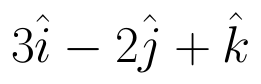from the plane 3x + y - z + 2+0 measured parallel to the line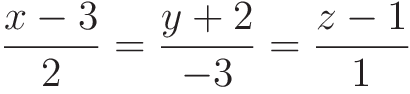Also, find the foot of the perpendicular from the given point upon the given plane. (View Answer Video)

Question 5 : Find the vector equation of the plane with intercepts 3, -4 and 2 on x, y and z-axis respectively. (View Answer Video)

### Differential Equations

Question 1 : If y(x) is a solution of the differential equation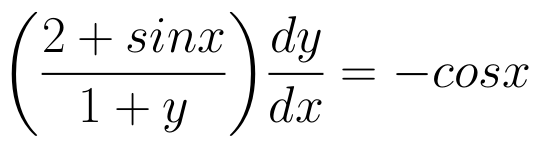and y(0) = 1, then find the value of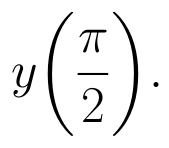(View Answer Video)

Question 2 : Find the particular solution of the differential equation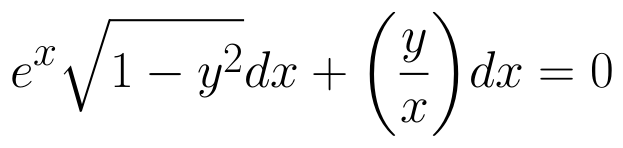given that y = 1 when x = 0. (View Answer Video)

Question 3 : Determine degree of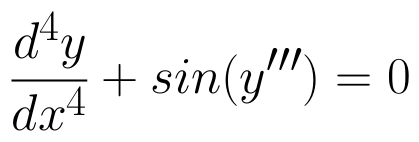(View Answer Video)

Question 4 : Solve the differential equation: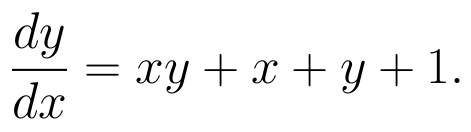(View Answer Video)

Question 5 : Solve the differential equation: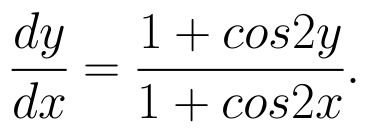(View Answer Video)

### Matrices

Question 1 : Given,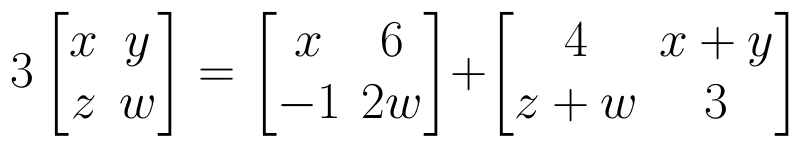, find the value of z. (View Answer Video)

Question 2 : If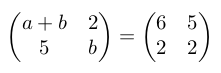, then find "a". (View Answer Video)

Question 3 : Find the value of x,  from the equation: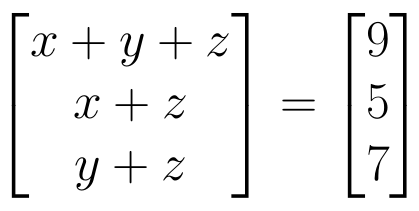. (View Answer Video)

Question 4 : Find the value of z,  from the equation:. (View Answer Video)

Question 5 :  Find the value of t, if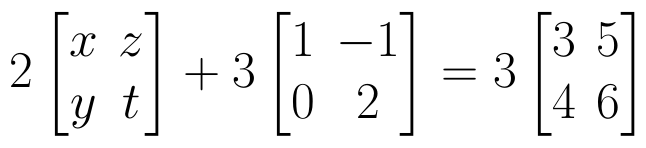(View Answer Video)

### Probability

Question 1 : A bag A contains 4 black and 6 red balls and bag B 7 black and 3 red balls. A die is thrown. If 1 or 2 appears on it, then bag A is chosen, otherwise bag B. It two balls are drawn at random (without replacement) from the selected bag, find the probability of one of them being red and another black.        (View Answer Video)

Question 2 :  Three numbers are selected at random (without replacement ) from first six positive integers. Let X denote the largest of the three numbers obtained. Find the probability distribution of X. Also, find the mean and variance of the distribution.  (View Answer Video)

Question 3 :  Four cards are drawn successively with replacement from a well shuffled deck of 52 cards. What is the probability that:
All the four cards are spades?